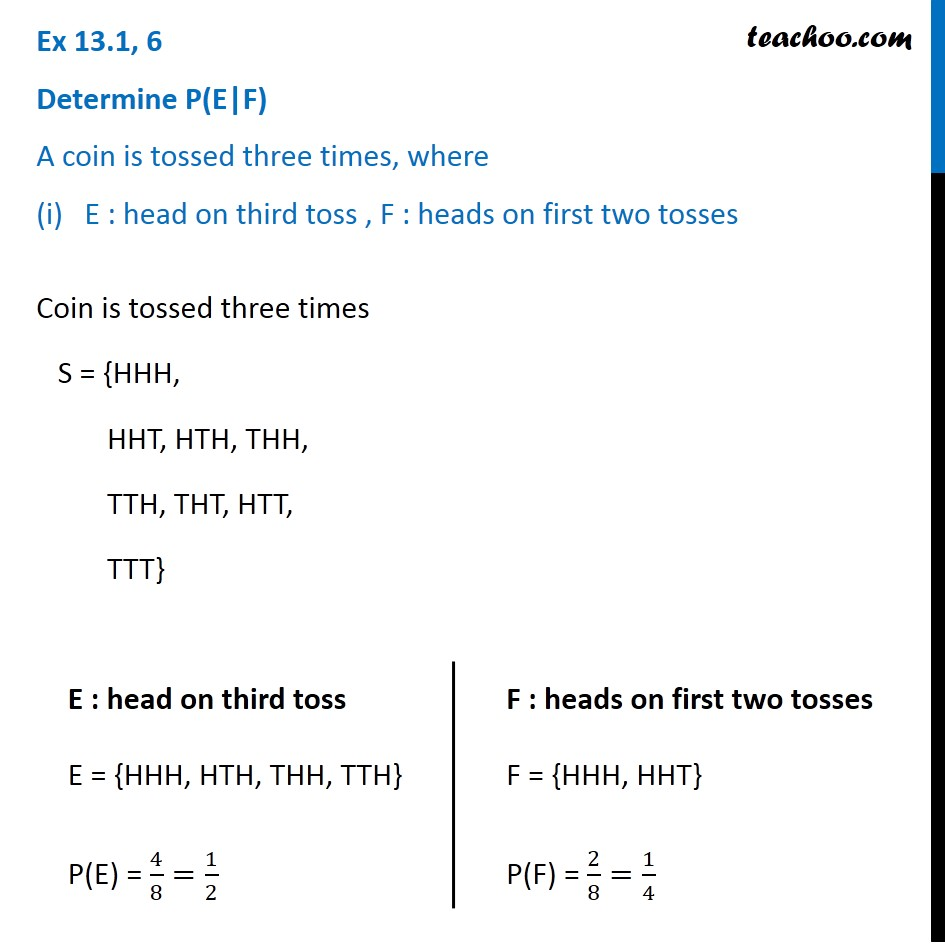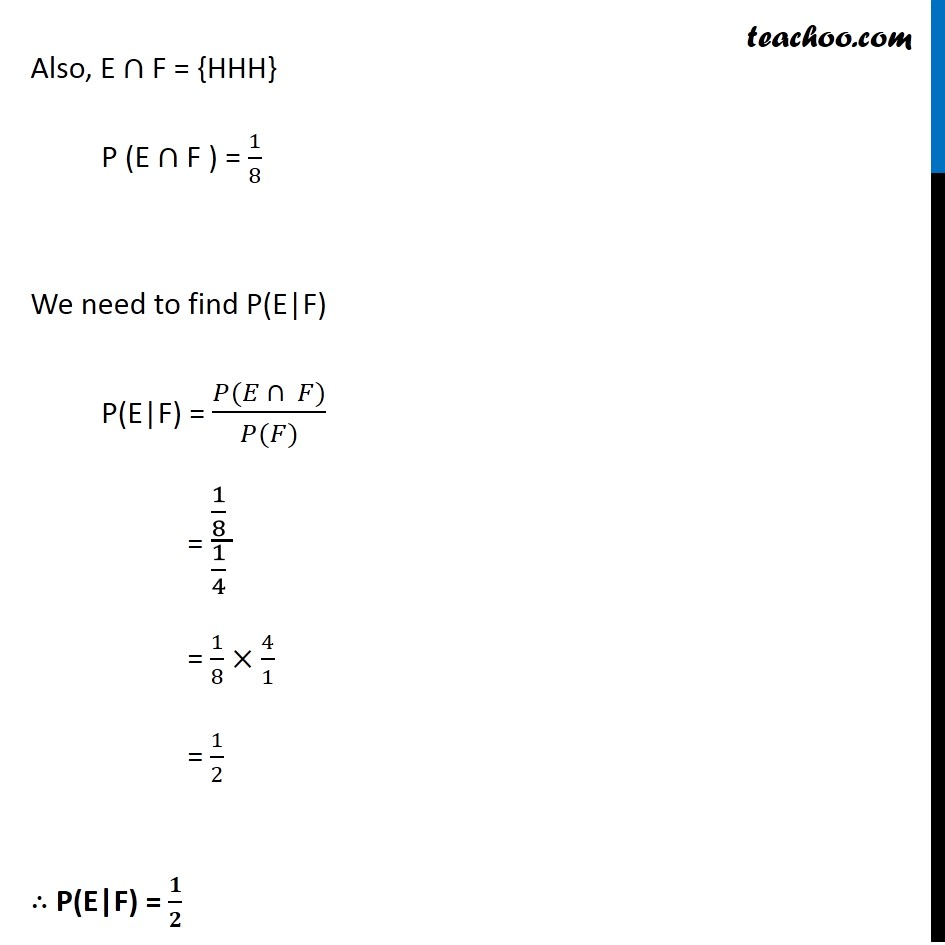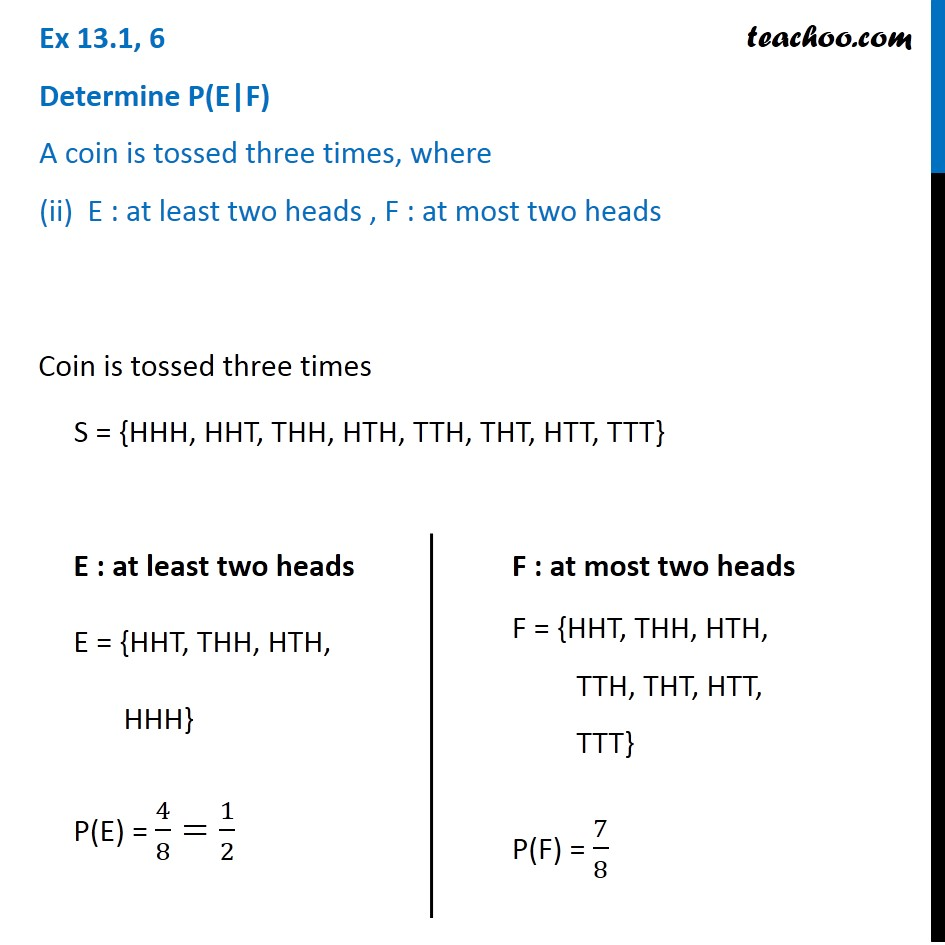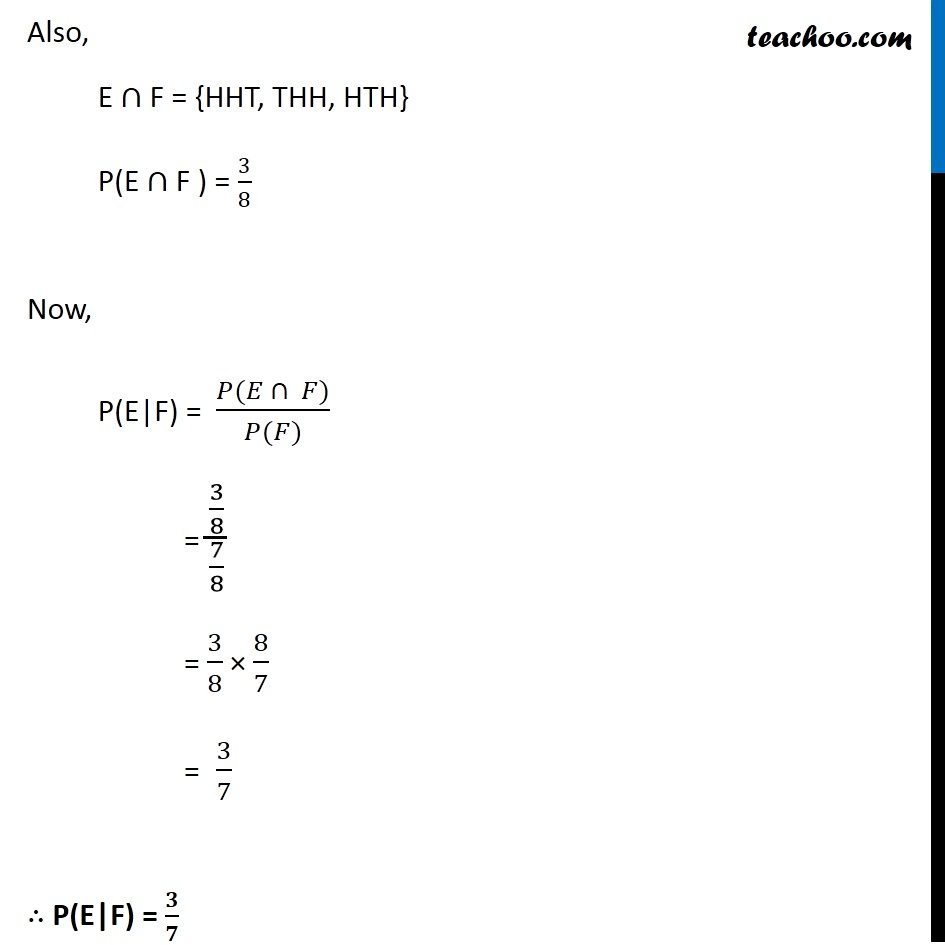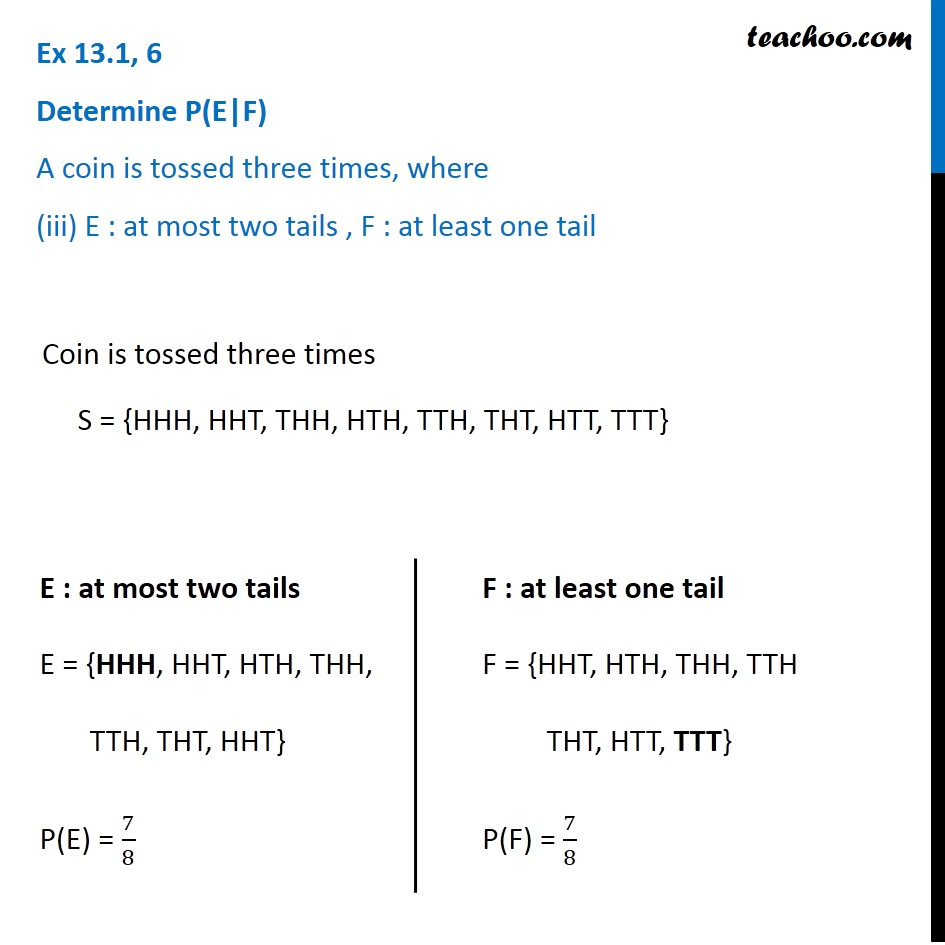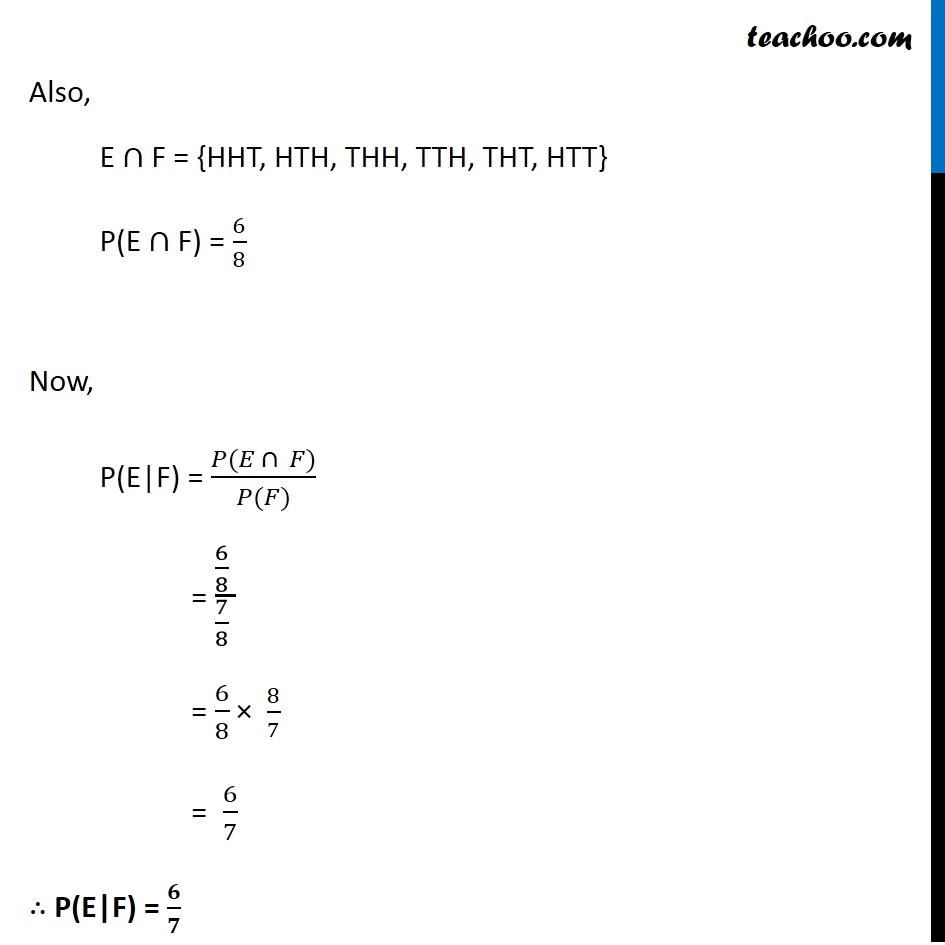Subscribe to our Youtube Channel - https://you.tube/teachoo

1. Chapter 13 Class 12 Probability
2. Serial order wise
3. Ex 13.1

Transcript

Ex 13.1, 6 ﻿Determine P(E|F) A coin is tossed three times, where (i) E : head on third toss , F : heads on first two tossesCoin is tossed three times S = {HHH, HHT, HTH, THH, TTH, THT, HTT, TTT} E : head on third toss E = {HHH, HTH, THH, TTH} P(E) = 4/8=1/2 F : heads on first two tosses F = {HHH, HHT} P(F) = 2/8=1/4 Also, E ∩ F = {HHH} P (E ∩ F ) = 1/8 We need to find P(E|F) P(E|F) = (𝑃(𝐸 ∩ 𝐹))/(𝑃(𝐹)) = (1/8 )/(1/4 ) = 1/8×4/1 = 1/2 ∴ P(E|F) = 𝟏/𝟐 Ex 13.1, 6 ﻿Determine P(E|F) A coin is tossed three times, where (ii) E : at least two heads , F : at most two heads Coin is tossed three times S = {HHH, HHT, THH, HTH, TTH, THT, HTT, TTT} Coin is tossed three times S = {HHH, HHT, THH, HTH, TTH, THT, HTT, TTT} E : at least two heads E = {HHT, THH, HTH, HHH} P(E) = 4/8=1/2 F : at most two heads F = {HHT, THH, HTH, TTH, THT, HTT, TTT} P(F) = 7/8 Also, E ∩ F = {HHT, THH, HTH} P(E ∩ F ) = 3/8 Now, P(E|F) = (𝑃(𝐸 ∩ 𝐹))/(𝑃(𝐹)) = (3/8)/(7/8) = 3/8 × 8/7 = 3/7 ∴ P(E|F) = 𝟑/𝟕 Ex 13.1, 6 ﻿Determine P(E|F) A coin is tossed three times, where (iii) E : at most two tails , F : at least one tail Coin is tossed three times S = {HHH, HHT, THH, HTH, TTH, THT, HTT, TTT} E : at most two tails E = {HHH, HHT, HTH, THH, TTH, THT, HHT} P(E) = 7/8 F : at least one tail F = {HHT, HTH, THH, TTH THT, HTT, TTT} P(F) = 7/8 Also, E ∩ F = {HHT, HTH, THH, TTH, THT, HTT} P(E ∩ F) = 6/8 Now, P(E|F) = (𝑃(𝐸 ∩ 𝐹))/(𝑃(𝐹)) = (6/8 )/(7/8 ) = 6/8 × 8/7 = 6/7 ∴ P(E|F) = 𝟔/𝟕

Ex 13.1